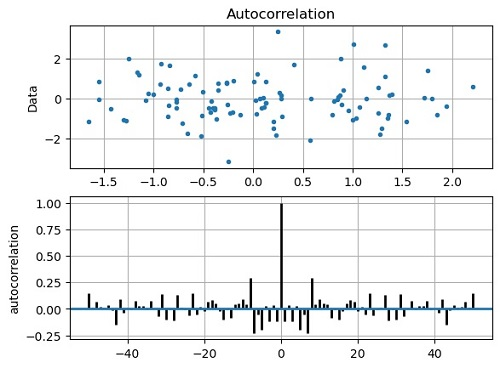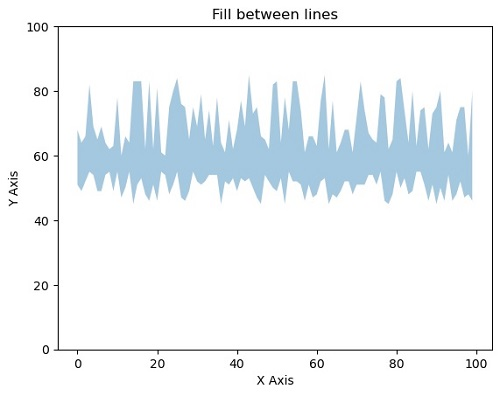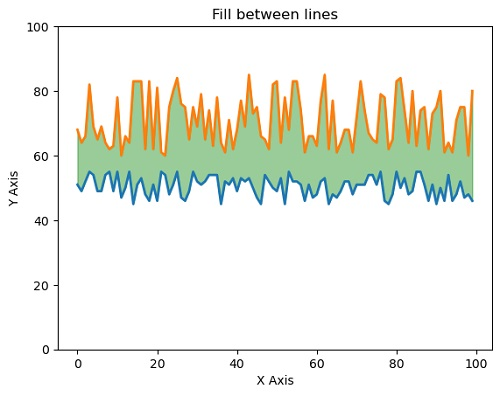# Python | Autocorrelation Plot using Matplotlib

matplotlib.pyplot.acorr(): In this tutorial, we are going to learn how to plot autocorrelation in python using matplotlib?
Submitted by Anuj Singh, on July 24, 2020

In some of the applications, we need to plot autocorrelation and therefore, matplotlib has an inbuilt defined function for our desired operation i.e. matplotlib.pyplot.acorr(). Following are the examples showing the implementation of matplotlib.pyplot.acrr().

Illustration:

```matplotlib.pyplot.acorr(x, usevlines=True, normed=True, maxlags=50, lw=2)
``````matplotlib.pyplot.fill_between(x, y, yy, alpha=0.4)
#Filling the area between two lines completely with opaque ratio 0.4
``````matplotlib.pyplot.plot(x,y, yy, linewidth=2.0)
matplotlib.pyplot.fill_between(x, y, yy, color='green', alpha=0.4)
#Filling the area between two lines completely
#in addition to show the lines as well.
```## Python code for autocorrelation plot using matplotlib

```import matplotlib.pyplot as plt
import numpy as np

x, y = np.random.randn(2, 100)

plt.figure()
plt.subplot(211)
plt.plot(x, y, '.')
plt.title('Autocorrelation')
plt.ylabel('Data')
plt.grid(True)

plt.subplot(212)
plt.acorr(x, usevlines=True, normed=True, maxlags=50, lw=2)
plt.ylabel('autocorrelation')
plt.grid(True)

plt.show()
```

Output:

```Output is as figure
```

Languages: » C » C++ » C++ STL » Java » Data Structure » C#.Net » Android » Kotlin » SQL
Web Technologies: » PHP » Python » JavaScript » CSS » Ajax » Node.js » Web programming/HTML
Solved programs: » C » C++ » DS » Java » C#
Aptitude que. & ans.: » C » C++ » Java » DBMS
Interview que. & ans.: » C » Embedded C » Java » SEO » HR
CS Subjects: » CS Basics » O.S. » Networks » DBMS » Embedded Systems » Cloud Computing
» Machine learning » CS Organizations » Linux » DOS
More: » Articles » Puzzles » News/Updates